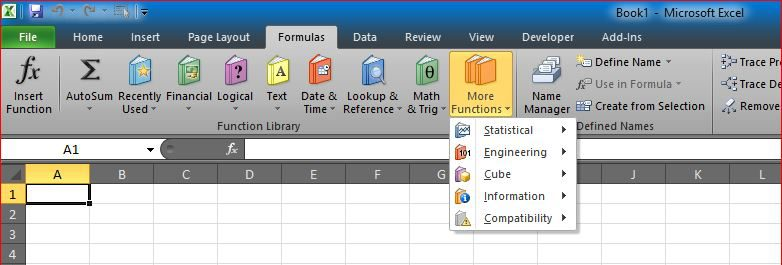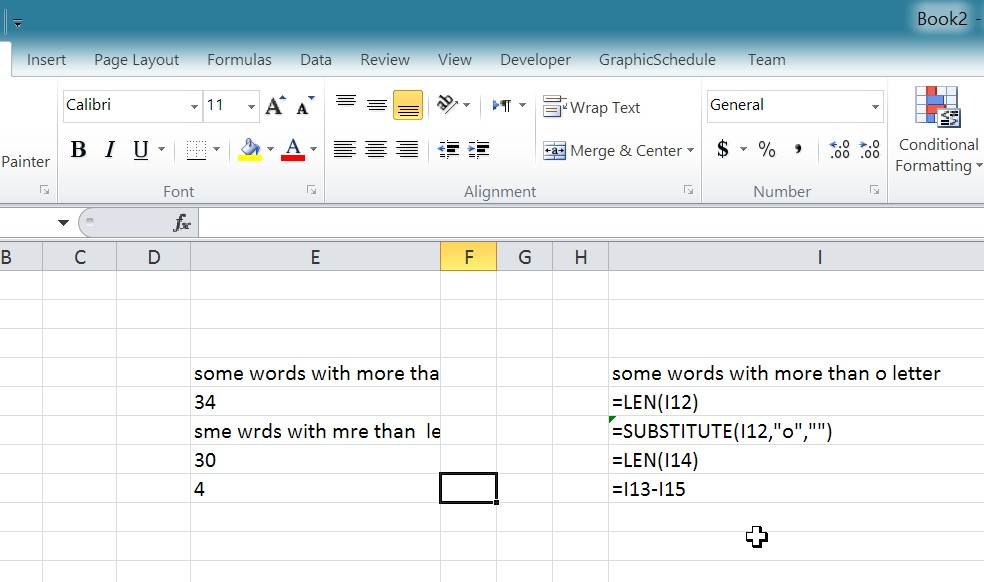# Count how many times letter foundFound this in an old file while doing some digital cleaning

This will show you how you can easily count how many times a letter (or any string) into a cell.
So the main formula doing that is …

`=LEN(I12)-LEN(SUBSTITUTE(I12,"o",""))`

Of course, this assumes you are looking to find how many the letter “O” is found. one letter

If you are looking to count how many times a word (more than 1 letter) you need to adjust that

`=LEN(I12)-(LEN(SUBSTITUTE(I12,"om","")/2))`

Too count for “OM”, notice that the 2 at end is the number of characters you are looking to count for

You may sure adjust that to cover more options, or dynamic, the idea is as below…

We count the length of the string, then we subtract it from the length of it after we replace all strings found

Does that make sense?